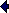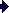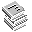Calculator for round wire helical springs Diameter of spring wire d 10-3 m Mean coil diameter D 10-3 m Number of active coils n -Shear modulus G = E / ( 2 ( 1+v ) ) 109 Pa Spring force F N Spring outer diameter Dout = D + d 10-3 m Spring radius r = D / 2 10-3 m Spring length closed (solid) Lc = n d 10-3 m Spring deflection f 10-3 m Energy stored W = F f / 2 J Spring stiffness k = dF / df = F / f 103 N/m Spring length free L0 > Lc + f 10-3 m Pitch of lead s = L0 / n 10-3 mShear stress τ 106 Pawww.werktuigbouw.nl